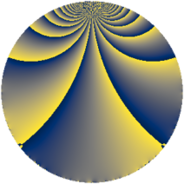# Properties

 Label 105.2.pLevel $105$ Weight $2$ Character orbit 105.p Rep. character $\chi_{105}(59,\cdot)$ Character field $\Q(\zeta_{6})$ Dimension $24$ Newform subspaces $1$ Sturm bound $32$ Trace bound $0$

# Related objects

## Defining parameters

 Level: $$N$$ $$=$$ $$105 = 3 \cdot 5 \cdot 7$$ Weight: $$k$$ $$=$$ $$2$$ Character orbit: $$[\chi]$$ $$=$$ 105.p (of order $$6$$ and degree $$2$$) Character conductor: $$\operatorname{cond}(\chi)$$ $$=$$ $$105$$ Character field: $$\Q(\zeta_{6})$$ Newform subspaces: $$1$$ Sturm bound: $$32$$ Trace bound: $$0$$

## Dimensions

The following table gives the dimensions of various subspaces of $$M_{2}(105, [\chi])$$.

Total New Old
Modular forms 40 40 0
Cusp forms 24 24 0
Eisenstein series 16 16 0

## Trace form

 $$24q - 12q^{4} - 6q^{9} + O(q^{10})$$ $$24q - 12q^{4} - 6q^{9} - 24q^{15} - 12q^{16} - 6q^{21} + 18q^{24} - 12q^{25} + 18q^{30} + 84q^{36} - 12q^{39} - 72q^{40} - 18q^{45} + 36q^{46} - 12q^{49} - 12q^{51} - 36q^{54} + 12q^{60} + 36q^{61} + 24q^{64} - 72q^{66} + 108q^{70} + 72q^{75} + 48q^{79} - 6q^{81} + 48q^{84} + 48q^{85} - 96q^{91} - 72q^{94} - 90q^{96} - 48q^{99} + O(q^{100})$$

## Decomposition of $$S_{2}^{\mathrm{new}}(105, [\chi])$$ into newform subspaces

Label Dim. $$A$$ Field CM Traces $q$-expansion
$$a_2$$ $$a_3$$ $$a_5$$ $$a_7$$
105.2.p.a $$24$$ $$0.838$$ None $$0$$ $$0$$ $$0$$ $$0$$